NCERT Solutions for Class 9 Maths Chapter 7 Heron’s Formula Ex 7.2 are part of NCERT Solutions for Class 9 Maths. Here we have given NCERT Solutions for Class 9 Maths Chapter 7 Heron’s Formula Ex 7.2.

 Board CBSE Textbook NCERT Class Class 9 Subject Maths Chapter Chapter 7 Chapter Name Heron’s Formula Exercise Ex 7.2 Number of Questions Solved 9 Category NCERT Solutions

## NCERT Solutions for Class 9 Maths Chapter 7 Heron’s Formula Ex 7.2

Question 1.
A park, in the shape of a quadrilateral ABCD, has ∠C = 90°, AB = 9m,BC = 12m,CD = 5m and AD = 8 m.
How much area does it occupy?
Solution:
In right ∆ BCD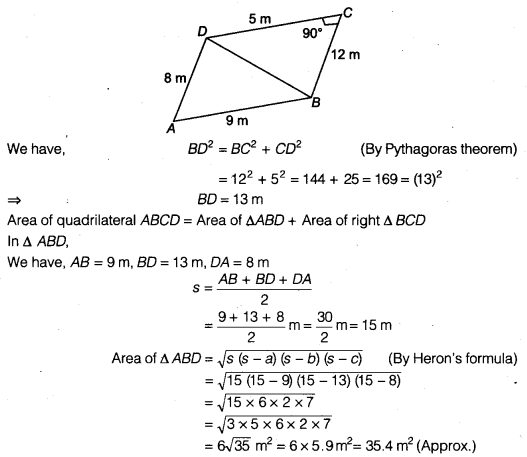Question 2.
Find the area of a quadrilateral ABCD in which AB = 3 cm, BC = 4 cm, CD = 4 cm, DA = 5 cm and AC = 5 cm.
Solution:
Area of quadrilateral ABCD = Area of ∆ABC+ Area of ∆ACD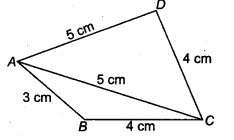In ∆ ABC,
We have, AB = 3 cm, BC = 4 cm, CA = 5 cm
Therefore, AB2 + BC2 = 32 + 42 = 9 + 16 = 25 = (5)2 = CA2
Hence, ∆ ABC is a right triangleQuestion 3.
Radha made a picture of an aeroplane with coloured paper as shown in figure. Find the total area of the paper used.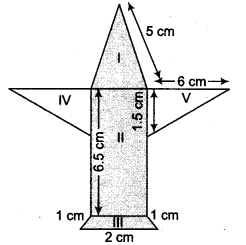Solution:
For part I
It is a triangle with sides 5 cm, 5cm and 1cm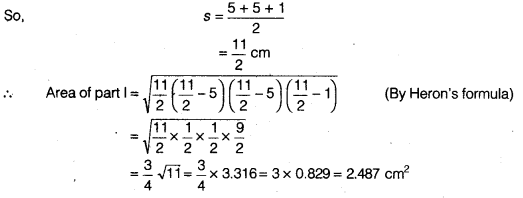For part II
It is a rectangle with sides 6.5 cm and 1 cm
∴ Area of part II = 6.5 x 1
(∵ Area of rectangle = Length x Breadth)
= 6.5 cm2

For part III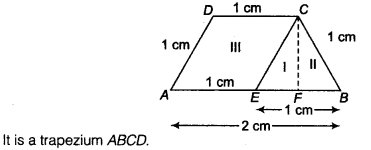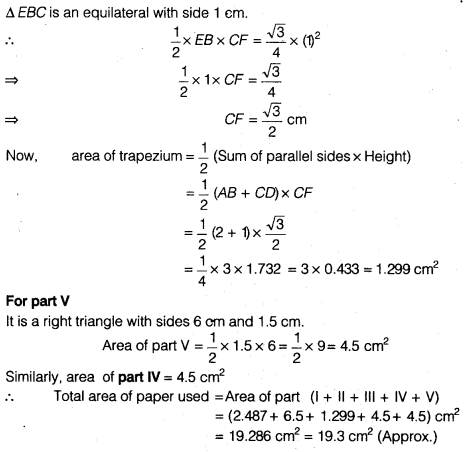Question 4.
A triangle and a parallelogram have the same base and the same area. If the sides of the triangle are 26 cm, 28 cm and 30 cm, and the parallelogram stands on the base 28 cm, find the height of the parallelogram
Solution:
Let ABC be a triangle with sides
AB = 26 cm, BC = 28 cm, CA = 30 cmWe know that,
Area of parallelogram = Base x Height …(i)
We have,
Area of parallelogram = Area of ∆ ABC (Given)
= 336 cm2
From Eq. (i), we have
Base x Height = 336
⇒ 28 x Height = 336
⇒ Height = $$\frac { 336 }{ 28 }$$
⇒ Height =12cm

Question 5.
A rhombus shaped field has green grass for 18 cows to graze. If each side of the rhombus is 30 m and its longer diagonal is 48 m, how much area of grass field will each cow be getting?
Solution:
Let ABCD be a rhombus.
Area of the rhombus ABCD = 2x area of ∆ ABD …(i)
(Since, in a rhombus diagonals divides two equal parts)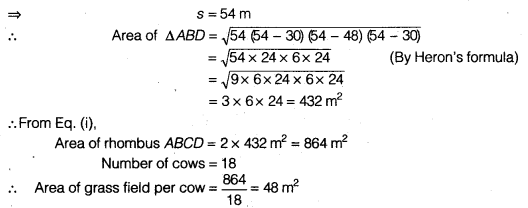Question 6.
An umbrella is made by stitching 10 triangular pieces of cloth of two different colours (see figure), each piece measuring 20 cm, 50 cm and 50 cm. How much cloth of each colour is required for the umbrella?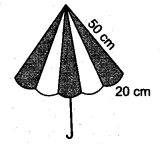Solution:
In an umbrella, each triangular piece is an isosceles triangle with sides 50 cm, 50 cm, 20 cm.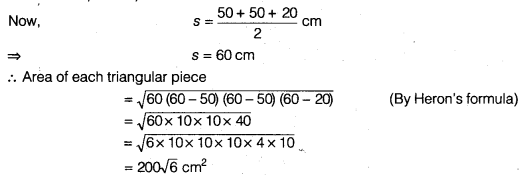Since, there are 10 triangular piece, in those of them 5.5 are of different colours.
Hence, total area of cloth of each colour = 5 x 200√6 cm2 = 1000√6 cm2

Question 7.
A kite in the shape of a square with a diagonal 32 cm and an isosceles triangle of base 8 cm and sides 6 cm each is to be made of three different shades as shown in figure. How much paper of each shade has been used in it?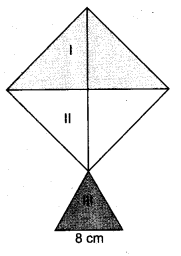Solution:
Since, the kite is in the shape of a square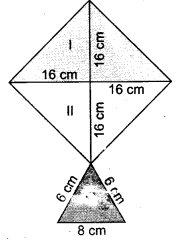Each diagonal of square =32 cm (Given)
We know that, the diagonals of a square bisect each other at right angle.Hence, paper of I colour has been used = 256 cm2
Paper of II colour has been used = 256 cm2
Paper of III colour has been used = 17.92 cm2

Question 8.
A floral design on a floor is made up of 16 tiles which are triangular, the sides of the triangle being 9 cm, 28 cm and 35 cm (see figure). Find the cost of polishing the tiles at the rate of 50 paise per cm .Solution:
Given, the sides of a triangular tiles are 9 cm, 28 cm and 35 cm.
For each triangular tile, we have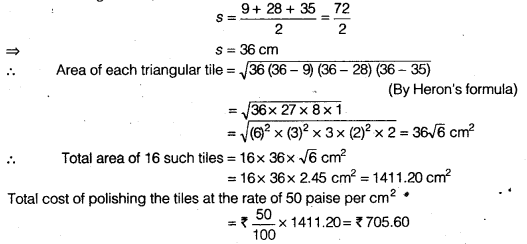Question 9.
A field is in the shape of a trapezium whose parallel sides are 25 m and 10 m. The non-parallel sides are 14 m and 13 m. Find the area of the field.
Solution:
Here, ABCD is a trapezium and AB || DC.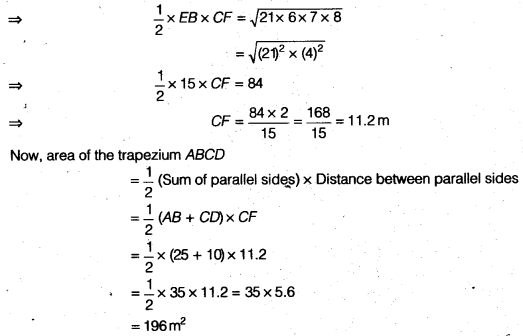We hope the NCERT Solutions for Class 9 Maths Chapter 7 Heron’s Formula Ex 7.2 help you. If you have any query regarding NCERT Solutions for Class 9 Maths Chapter 7 Heron’s Formula Ex 7.2, drop a comment below and we will get back to you at the earliest.